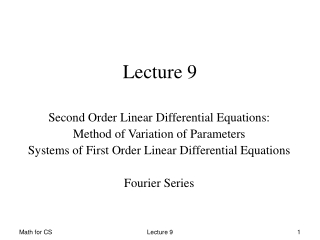DownloadDownload PresentationLecture 9

# Lecture 9

Télécharger la présentation## Lecture 9

- - - - - - - - - - - - - - - - - - - - - - - - - - - E N D - - - - - - - - - - - - - - - - - - - - - - - - - - -
##### Presentation Transcript

1. Lecture 9 Second Order Linear Differential Equations: Method of Variation of Parameters Systems of First Order Linear Differential Equations Fourier Series Lecture 9

2. The Linear Case A linear second order differential equations is written as associate the so called associated homogeneous equation For the study of these equations we consider the explicit ones given by A linear second order differential equations is written as associate the so called associated homogeneous equation For the study of these equations we consider the explicit ones given by (NH) (H) Lecture 9

3. Solution of the Linear Case where p(x) = b(x)/a(x), q(x) = c(x)/a(x) and g(x) = d(x)/a(x). If p(x), q(x) and g(x) are defined and continuous on the interval I, then the IVP has a unique solution defined on I. The general solution to the equation (NH) is given by where is the general solution to the homogeneous associated equation (H); is a particular solution to the equation (NH). Lecture 9

4. Homogeneous Linear Equations with Constant Coefficients Consider a nonhomogeneous equation with constant coefficients: where a, b and c are constants and g(x) is an exponent, polynom or harmonic function (eax; a0xn+…an; sin(wx) or cos(wx) ) or their product. This type of equation is very useful in many applied problems (physics, electrical engineering, etc..). Then one should make an intelligent guess about the form of the solution, up to the constant multipliers, and then substitute this guess into the equation to calculate the values of the multipliers. Lecture 9

5. The Method of variation of Parameters Consider a nonhomogeneous equation: Suppose y1 and y2 are linearly independent solutions of the homogeneous equation. Then the general solution of (2) Is c1y1+c2y2the method of variation of parameters involves replacement of constants c1 and c2 by functions u1 and u2. We then substitute y= u1y1+u2y2 into (1) to determine the functions. (1) (2) Lecture 9

6. The Method of variation of Parameters (3) We obtain: Since we are looking for a particular solution of second order equation, there are two arbitrary parameters or constraints. The first will be to satisfy (1), the second: Then Substitution into (1) yields Since y1 and y2 were solutions of homogeneous equations, the terms in the parenthesis vanish, and we remain with: (4) (5) Lecture 9

7. The Method of variation of Parameters Equations (4) and (5) together yield a system: Solving this system gives: Combining it all, we get: Lecture 9

8. Systems of the First Order LDE 1 Consider the system of first order linear differential equations with constant coefficients: The scalar differential equation of order n can be brought to the form of such linear system: We introduce the n dimensional vector: (2.1) (2.2) (2.3) Lecture 9

9. Systems of the First Order LDE 2 And x satisfies n-1 additional equations: For i=1,…n-1. Now we can write the original equation (2.2) as follows: ,where the matrix A and vector b are defined as: A is called a companion matrix of equation (2.2) (2.5) (2.6) Lecture 9

10. Systems of the First Order LDE 3 The general solution to the system, as we know is x(t)=xh(t)+xp(t), where xh(t) is the solution of homogeneous system: x’=Ax x(0)=x0 and xp(t) is a particular solution of non-homogeneous system. x’=Ax+b(t) x(0)=x0 The two solutions, xh(t) and xp(t) can be written by means of the matrix exponential, introduced in the following. The usual, scalar exponent can be defined via its Taylor series: Lecture 9

11. Systems of the First Order LDE 4 In the same way the exponent of a matrix A is defined by: The exponent of the matrix obeys the main property of exponential function: Thus, for any vector w, the function xh (t) = eAtw satisfies the homogeneous differential system x’h = Axh By using the initial values (2.1) we obtain xh(t)= eAtx(0) (2.7) (2.8) Lecture 9

12. Systems of the First Order LDE 5 (2.9) The particular solution is obtained as what can be verified: Equations (2.8-9) describe the solution of linear system (2.1;2.5), however we do not know how to compute the matrix exponential. Straightforward way (2.7) requires many terms for good approximation. Consider now the case when A has n linearly independent eigenvectors q1,…,qn. Let Q=[q1,…,qn] Lecture 9

13. Systems of the First Order LDE 6 Then and the homogeneous system can be rewritten as follows: The last equation represents n uncoupled, homogeneous, differential equations yi=λiyi And its homogeneous solution is yi=eΛty(0), where Using the relations x=Qy, y0=Q-1x0 we obtain: Lecture 9

14. Fourier Series In the same way a vector can be decomposed and represented in a basis of vectors, a function can be represented in a basis of functions. Similarly to geometry or linear algebra, an appropriate basis can make the problem easier. For harmonic problems, which compose many, if not most, mathematical and physical problems the Fourier Series is an invaluable tool. In the following, we will consider only the 2π periodic functions. First, let us consider the representation of the function in some orthonormal basis: Orthonormality of the basis means that the scalar product of different basis functions equals 0, and of the function with itself equals 1: (1) (2) Lecture 9

15. Fourier Series How to find cn in (1) ? Let us multiply (1) by φn(x): Here we used the orthonormality of the basis {φn(x)}. If the basis functions are ,then the series With an and bn generated by (3) is called a Fourier series, generated by f. (3) Lecture 9

16. Partial sums. Gibbs Phenomena Lecture 9